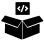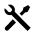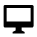Welcome to Ray!

# Welcome to Ray!

Ray is an open-source unified framework for scaling AI and Python applications. It provides the compute layer for parallel processing so that you don’t need to be a distributed systems expert.

## Scaling with Ray

``````
from typing import Dict
import numpy as np

import ray

# Step 1: Create a Ray Dataset from in-memory Numpy arrays.
ds = ray.data.from_numpy(np.asarray(["Complete this", "for me"]))

# Step 2: Define a Predictor class for inference.
class HuggingFacePredictor:
def __init__(self):
from transformers import pipeline
# Initialize a pre-trained GPT2 Huggingface pipeline.
self.model = pipeline("text-generation", model="gpt2")

# Logic for inference on 1 batch of data.
def __call__(self, batch: Dict[str, np.ndarray]) -> Dict[str, list]:
# Get the predictions from the input batch.
predictions = self.model(list(batch["data"]), max_length=20, num_return_sequences=1)
# `predictions` is a list of length-one lists. For example:
# [[{'generated_text': 'output_1'}], ..., [{'generated_text': 'output_2'}]]
# Modify the output to get it into the following format instead:
# ['output_1', 'output_2']
batch["output"] = [sequences["generated_text"] for sequences in predictions]
return batch

# Use 2 parallel actors for inference. Each actor predicts on a
# different partition of data.
scale = ray.data.ActorPoolStrategy(size=2)
# Step 3: Map the Predictor over the Dataset to get predictions.
predictions = ds.map_batches(HuggingFacePredictor, compute=scale)
# Step 4: Show one prediction output.
predictions.show(limit=1)

``````
``````
from ray.air.config import ScalingConfig
from ray.train.torch import TorchTrainer

# Step 1: setup PyTorch model training as you normally would
def train_loop_per_worker():
model = ...
train_dataset = ...
for epoch in range(num_epochs):
...  # model training logic

# Step 2: setup Ray's PyTorch Trainer to run on 32 GPUs
trainer = TorchTrainer(
train_loop_per_worker=train_loop_per_worker,
scaling_config=ScalingConfig(num_workers=32, use_gpu=True),
datasets={"train": train_dataset},
)

# Step 3: run distributed model training on 32 GPUs
result = trainer.fit()
``````
``````
from ray import tune
from ray.air.config import ScalingConfig
from ray.train.lightgbm import LightGBMTrainer

train_dataset, eval_dataset = ...

# Step 1: setup Ray's LightGBM Trainer to train on 64 CPUs
trainer = LightGBMTrainer(
...
scaling_config=ScalingConfig(num_workers=64),
datasets={"train": train_dataset, "eval": eval_dataset},
)

# Step 2: setup Ray Tuner to run 1000 trials
tuner = tune.Tuner(
trainer=trainer,
param_space=hyper_param_space,
tune_config=tune.TuneConfig(num_sa
les=1000),
)

# Step 3: run distributed HPO with 1000 trials; each trial runs on 64 CPUs
result_grid = tuner.fit()

``````
``````
import pandas as pd

from ray import serve
from starlette.requests import Request

@serve.deployment(ray_actor_options={"num_gpus": 1})
class PredictDeployment:
def __init__(self, model_id: str, revision: str = None):
from transformers import AutoModelForCausalLM, AutoTokenizer
import torch

self.model = AutoModelForCausalLM.from_pretrained(
model_id,
…
)
self.tokenizer = AutoTokenizer.from_pretrained(model_id)

def generate(self, text: str) -> pd.DataFrame:
input_ids = self.tokenizer(text, return_tensors="pt").input_ids.to(
self.model.device
)

gen_tokens = self.model.generate(
input_ids,
…
)
return pd.DataFrame(
self.tokenizer.batch_decode(gen_tokens), columns=["responses"]
)

async def __call__(self, http_request: Request) -> str:
prompts: list[str] = await http_request.json()["prompts"]
return self.generate(prompts)

``````
``````
from ray.rllib.algorithms.ppo import PPOConfig

# Step 1: configure PPO to run 64 parallel workers to collect samples from the env.
ppo_config = (
PPOConfig()
.environment(env="Taxi-v3")
.rollouts(num_rollout_workers=64)
.framework("torch")
.training(model=rnn_lage)
)

# Step 2: build the PPO algorithm
ppo_algo = ppo_config.build()

# Step 3: train and evaluate PPO
for _ in range(5):
print(ppo_algo.train())

ppo_algo.evaluate()
``````

## Beyond the basics### Ray AI Runtime

Scale the entire ML pipeline from data ingest to model serving with high-level Python APIs that integrate with popular ecosystem frameworks.### Ray Core

Scale generic Python code with simple, foundational primitives that enable a high degree of control for building distributed applications or custom platforms.